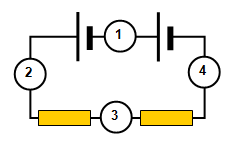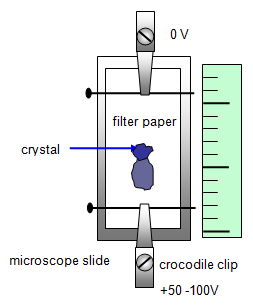## Flow of electrical charge

[Where needed take the charge on the electron to be – 1.6x10-19 C]

1. Explain the terms in the formula I = nAve

2. What happens to the drift velocity of the electron cloud in a metal wire if the current through it increases?

3. There are 8x1028 electrons per cubic metre in a sample of copper. Calculate:
(a) their drift velocity when a current of 0.5 A flows in a wire of diameter 0.28 mm.
(b) the number of electrons passing any point in the wire in one minute

4. Calculate the free electron concentration in silver if the drift velocity of the free electron cloud is 0.03 mms-1 in a silver wire of cross sectional area 1.5x10-8 m2 when a current of 4 mA flows through it.5. This question is about the circuit shown in the diagram on the right. If the reading on ammeter 1 is 0.3 A calculate the following:
(a) the reading on ammeter 2
(b) the reading on ammeter 3
(c) the reading on ammeter 4
(d) the charge passing through ammeter 2 in 15 s
(e) the number of electrons passing through ammeter 4 in 20 s

6. A table tennis ball oscillates between two charged vertical metal plates. A sensitive ammeter connected between the plates records a current of 3 μA when the period of oscillation of the ball is 2 s. Calculate: (a) the charge carried between the plates by the ball in each oscillation (b) the number of electrons carried between the plates by the ball in each oscillation

7. In a television tube 0.25 m long the electron velocity is 5x107 ms-1. If the current in the tube is 1.5 mA calculate:
(a) the number of electrons reaching the screen every second
(b) the number of electrons in 1 cm of the beam8. The diagram shows the experimental arrangement of an experiment to find the velocity of charged ions.
It shows their movement during 50 s. Calculate:
(a) the ion drift velocity
(b) the charge reaching the positive electrode per second if the current is 1.25 mA
(c) the number of ions reaching the positive electrode per second if there are each doubly charged